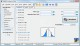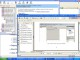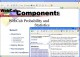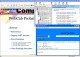ESBPDF Analysis - Probability Software 2.4.1 Probability Analysis Software for Windows that is easy to use. SharewareDomino Probability 1.0 Students are shown a set of dominos and asked the probability of a certain outcome, such as picking a domino whose two numbers sum to eight. FreewareWebCab Probability and Stat for Delphi 3.6 Statistics, Discrete Prob, Distributions, Hypo. testing, Correlation,Regression DemoWebCab Probability and Stat (J2EE Ed.) 3.6 Statistics, Discrete Prob, Distributions, Hypo. testing, Correlation,Regression DemoProbability Calc 1.0 Probability Calc offers you an application that calculates the probability of z, t, F, and chi-square values. FreewareWebCab Probability and Stat (J2SE Ed.) 3.6 Statistics, Discrete Prob, Distributions, Hypo. testing, Correlation,Regression DemoWebCab Probability and Stat for .NET 3.3 Statistics, Discrete Prob, Distributions, Hypo. testing, Correlation,Regression DemoCosmic Debris 2.0 Cosmic Debris is an Aurora Borealis (AKA the Northern Lights) monitor menu item. FreewareWebCab Probability and Stat (J2SE Ed.) 3.3 Statistics, Discrete Prob, Distributions, Hypo. testing, Correlation,Regression DemoWebCab Probability and Stat for .NET 3.6 Statistics, Discrete Prob, Distributions, Hypo. testing, Correlation,Regression Demo